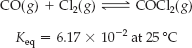×
Get Full Access to Introductory Chemistry - 5 Edition - Chapter 15 - Problem 93p
Get Full Access to Introductory Chemistry - 5 Edition - Chapter 15 - Problem 93p

×

# Consider the reaction. A 3.67-L flask containing an equilibrium reaction mixture hasISBN: 9780321910295 34

## Solution for problem 93P Chapter 15

Introductory Chemistry | 5th Edition

• Textbook Solutions
• 2901 Step-by-step solutions solved by professors and subject experts
• Get 24/7 help from StudySoup virtual teaching assistantsIntroductory Chemistry | 5th Edition

4 5 1 412 Reviews
20
0
Problem 93P

Problem 93P

Consider the reaction.A 3.67-L flask containing an equilibrium reaction mixture has [H2] = 0.104 M and [I2] = 0.0202 M. What mass of HI in grams is in the equilibrium mixture?

Step-by-Step Solution:
Step 1 of 3

Chapter 3: Composition of Substances and Solutions Molecular and Formula Mass  The molecular mass is the mass in atomic mass units (amu) of an individual molecule. o To calculate the molecular mass, multiply the atomic mass for each element in a molecule by the number of atoms of that element and then total the masses. Example: Molecular Mass of HOH= 2(atomic mass of H) +atomic mass of O =2(1.008)+16.00amu=18.02 amu  Molecular weight is an average molecular mass, determined by the periodic table atomic masses, which are average atomic masses. Formula Mass  Formula Mass- a sum of the atomic weights of the

Step 2 of 3

Step 3 of 3

##### ISBN: 9780321910295

Unlock Textbook Solution